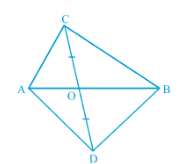Q

# In Fig. 9 24, ABC and ABD are two triangles on the same base AB. If line- segment CD is bisected by AB at O, show that ar (ABC) equals ar (ABD).

Q : 4    In Fig. ,  ABC and ABD are two triangles on the same base AB. If line- segment CD is bisected by AB at O, show that  .ViewsWe have, ABC and ABD on the same base AB. CD is bisected by AB at point O.
OC = OD
Now, in ACD, AO is median
ar (AOC) = ar (AOD)..........(i)

Similarly, in BCD, BO is the median
ar (BOC) = ar (BOD)............(ii)

Adding equation (i) and eq (ii), we get

ar (AOC) + ar (BOC) =  ar (AOD) + ar (BOD)

Hence proved.

Exams
Articles
Questions